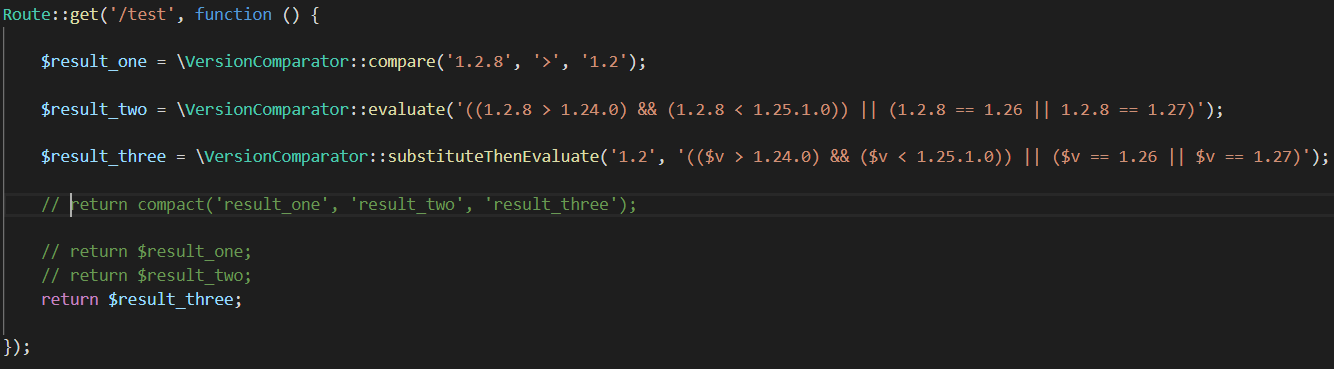## someshwer/version-comparison

This Laravel package compares two version strings and gives the Boolean result. This package also resolves version expressions like ((\$v > 1.24.0) && (\$v < 1.25.1.0)) || (\$v == 1.26 || \$v == 1.27) where \$v must be substituted with the version number to be compared. Hence the package can be used for...

##### Details

Homepage

Source

Issues

4

1

1

0

2.1.1 2018-10-18 12:59 UTC

# README

This Laravel package compares two version strings and gives the Boolean result. This package also resolves version expressions like ((\$v > 1.24.0) && (\$v < 1.25.1.0)) || (\$v == 1.26 || \$v == 1.27) where \$v must be substituted with the version number to be compared. Hence the package can be used for version expressions evaluation.

# Installation

To download the latest version, type the following line in terminal and then press enter. (Current stable version of this package is 2.1 LTS)

``````composer require someshwer/version-comparison
``````

And then add the following service provider to providers array in config/app.php configuration file.

``````Ex: 'providers' => [
... ,
... ,
Someshwer\VersionComparison\VersionComparisonServiceProvider::class
];
``````

And add the following alias to aliases array in config/app.php configuration file.

``````Ex: 'aliases' => [
... ,
.... ,
'VersionComparator' => Someshwer\VersionComparison\Facades\VersionComparator::class
];
``````

Now just simply publish the service provider by running following command in terminal. (Optional Step)

``````    \$ php artisan vendor:publish --provider="Someshwer\VersionComparison\VersionComparisonServiceProvider"

That's all! You are done with package installation...
``````

# Usage

1. VersionComparator::compare(): This method compares two version strings using specified operator and gives the Boolean result. It takes three parameters:
``````VersionComparator::compare(Version1 String, Operator String, Version2 String); // compare(Version1, Operator, Version2);

a. Version number (First Parameter)
b. Operator (Second Parameter)
c. Version number (Third Parameter)

Ex: VersionComparator::compare('1.2.5', '>', '1.2.2'); // Gives the Boolean result either TRUE or FALSE.
For this above example comparison, the result is TRUE.
``````
1. VersionComparator::evaluate(): This method usually evaluates version expression in the following form: (("1.2" > "1.24.0") && ("1.2" < "1.25.1.0")) || ("1.2" == "1.26" || "1.2" == "1.27") It takes only one argument that is expression of the above type.
``````VersionComparator::evaluate('Version Expression');

Ex: VersionComparator::evaluate('(("1.2" > "1.24.0") && ("1.2" < "1.25.1.0")) || ("1.2" == "1.26" || "1.2" == "1.27")');
// Gives Boolean result either TRUE or FALSE
``````
1. VersionComparator::substituteThenEvaluate(): This method usually evaluates version expressions of the following type: ((\$v > "1.24.0") && (\$v < "1.25.1.0")) || (\$v == "1.26" || \$v == "1.27") This method takes two parameters. They are: a. First one is the version number to be substituted in place of \$v in the expression. And b. The second one is the expression to be evaluated after \$v is substituted.

Note: \$v is the variable to be substituted with version number (First Parameter).

``````VersionComparator::substituteThenEvaluate('Version Number', 'Version Expression');

Ex: VersionComparator::substituteThenEvaluate('2.1.4', '((1.2 > 1.24.0) && (1.2 < 1.25.1.0)) || (1.2 == 1.26 || 1.2 == 1.27)');
Hence, in this example: \$v in the expression is replaced by '2.1.4'.

After successful evaluation it gives the Boolean result either TRUE or FLASE.
``````
``````Note: This package requires and depends on two other external third-party packages. They are:
1. composer/semver version 1.4.2 (https://github.com/composer/semver) and
2. symfony/expression-language version 4.1 (https://symfony.com/doc/current/components/expression_language.html).

You no need to manually install them in your project. They will be automatically downloaded to your vendor folder when ever
you install "someshwer/version-comparison" package by executing the following command in terminal.

-> composer require someshwer/version-comparison
``````

# Response

The response content must be json. The methods of this package almost always return the response in JSON format.

``````Example:

1. Sample success response:
{
status: "SUCCESS",
message: "Expression successfully evaluated!",
error_message: false,
}

2. Sample validation error response:
{
status: "ERROR",
error_type: "validation",
message: "Unable to evaluate the expression!",
error_message: "Invalid expression! Unexpected ")" around position 44 for expression `(('1.2' > '1.24.0')) && ('1.2' < '1.25.1.0')) || ('1.2' == '1.26' || '1.2' == '1.27')`. Check log for more info.",
}
``````

Note: If you want, you can also customize the response to the format which ever you want.!

# Code snippet for testing:

``````Route::get('/test', function () {

\$result_one = \VersionComparator::compare('1.2.8', '>', '1.2');

\$result_two = \VersionComparator::evaluate('((1.2.8 > 1.24.0) && (1.2.8 < 1.25.1.0)) || (1.2.8 == 1.26 || 1.2.8 == 1.27)');

\$result_three = \VersionComparator::substituteThenEvaluate('1.2', '((\$v > 1.24.0) && (\$v < 1.25.1.0)) || (\$v == 1.26 || \$v == 1.27)');

// return compact('result_one', 'result_two', 'result_three');

// return \$result_one;
// return \$result_two;
return \$result_three;

});
``````

If you want to test this package, copy and paste the above code in your web.php file and test this version-comparison/version-expression evaluation package.

# Screenshot of code snippet: